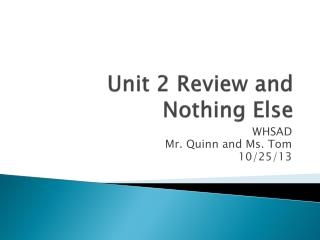DownloadDownload PresentationUnit 2 Review and Nothing Else

# Unit 2 Review and Nothing Else

Télécharger la présentation## Unit 2 Review and Nothing Else

- - - - - - - - - - - - - - - - - - - - - - - - - - - E N D - - - - - - - - - - - - - - - - - - - - - - - - - - -
##### Presentation Transcript

1. Unit 2 Review and Nothing Else WHSAD Mr. Quinn and Ms. Tom 10/25/13

2. Bohr Model: 2 Points • An orbital is defined as a region of the most probable location of • an electron • a neutron • a nucleus • a proton

3. Wave Model: 2 Points • Which phrase describes an atom? • a positively charged electron cloud surrounding a positively charged nucleus • a positively charged electron cloud surrounding a negatively charged nucleus • a negatively charged electron cloud surrounding a positively charged nucleus • a negatively charged electron cloud surrounding a negatively charged nucleus

4. Nuclear Chemistry: 2 Points • Which statement best describes gamma radiation? • It has a mass of 1 and a charge of 1. • It has a mass of 0 and a charge of –1. • It has a mass of 0 and a charge of 0. • It has a mass of 4 and a charge of +2.

5. Atomic Theory: 2 Points • Which statement about one atom of an element identifies the element? • The atom has 1 proton. • The atom has 2 neutrons. • The sum of the number of protons and neutrons in the atom is 3. • The difference between the number of neutrons and protons in the atom is 1.

6. Bohr Model: 4 Points • Which elements are present in this mixture? • E and D, only • E and G, only • D and G, only • D, E, and G

7. Wave Model: 4 Points • Which group of atomic models is listed in historical order from the earliest to the most recent? • hard-sphere model, wave-mechanical model, electron-shell model • hard-sphere model, electron-shell model, wave-mechanical model • electron-shell model, wave-mechanical model, hard-sphere model • electron-shell model, hard-sphere model, wave-mechanical model

8. Nuclear Chemistry: 4 Points • Radiation is spontaneously emitted from hydrogen-3 nuclei, but radiation is not spontaneously emitted from hydrogen-1 nuclei or hydrogen-2 nuclei. Which hydrogen nuclei are stable? • nuclei of H-1 and H-2, only • nuclei of H-1 and H-3 only • nuclei of H-2 and H-3 only • nuclei from H-1, H-2, and H-3

9. Atomic Theory: 4 Points • What is the charge of the nucleus of an oxygen atom? • 0 • –2 • +8 • +16

10. Bohr Model: 6 Points • Which atom in the ground state has an outermost electron with the most energy? • Cs • K • Li • Na

11. Wave Model: 6 Points • What is the total number of orbitals containing only one electron in an atom of nitrogen in the ground state? • 1 • 2 • 3 • 4

12. Nuclear Chemistry: 6 Points 1 2 3 4

13. Atomic Theory: 6 Points • As a result of the gold foil experiment, it was concluded that an atom • contains protons, neutrons, and electrons • contains a small, dense nucleus • has positrons and orbitals • is a hard, indivisible sphere

14. Bohr Model: 8 Points • Which electron configuration represents an atom of aluminum in an excited state? • 2-7-4 • 2-7-7 • 2-8-3 • 2-8-6

15. Wave Model: 8 Points • What is the total number of sublevels that contain electrons in the third principal energy level of a nickel atom in the ground state? • 1 • 2 • 3 • 4

16. Nuclear Chemistry: 8 Points • After decaying for 48 hours, 1/16 of the original mass of a radioisotope sample remains unchanged. What is the half-life of this radioisotope? • 3.0 h • 9.6 h • 12 h • 24 h

17. Atomic Theory: 8 Points • Atom X and atom Z are isotopes of the element • aluminum • Carbon • Magnesium • nitrogen

18. Bohr Model: 10 Points • Which atom in the ground state has a partially filled second electron shell? • hydrogen atom • lithium atom • potassium atom • sodium atom

19. Wave Model: 10 Points • An electron will emit energy in quanta when its energy state changes from 4p to • 5s • 5p • 3s • 6p

20. Nuclear Chemistry: 10 Points • An original sample of the radioisotope fluorine-21 had a mass of 80.0 milligrams. Only 20.0 milligrams of this original sample remain unchanged after 8.32 seconds. What is the half-life of fluorine-21? • 1.04s • 2.08s • 4.16s • 8.3s

21. Atomic Theory: 10 Points • The nucleus of an atom of cobalt-58 contains • 27 protons and 31 neutrons • 27 protons and 32 neutrons • 59 protons and 60 neutrons • 60 protons and 60 neutrons